Lesson 3: Numbers and Mathematical Calculations

In this lesson you will learn how to work with numbers and how to perform mathematical calculations. To begin, open Microsoft Excel.

Setting the Enter Key Direction

In Microsoft Excel, you can specify which direction the cursor moves when you press the Enter key. You can have the cursor move up, down, left, right, or not at all. Let’s make sure the cursor is set to move down when you press the Enter key.

1. Click on Tools, which is located on the Menu bar.
2. Press the down arrow key until Options is highlighted.
3. Press Enter.
4. Click on the Edit tab, if it is not in the front.
5. Make sure there is a checkmark in the "Move Selection after Enter" box.
6. If Down is not selected, click to open the Direction drop-down box. Click on Down.
7. Click on OK.
Making Numeric Entries

In Microsoft Excel, you can enter numbers and mathematical formulas into cells. When a number is entered into a cell, you can perform mathematical calculations such as addition, subtraction, multiplication, and division. When entering a mathematical formula, precede the formula with an equals sign. Use the following to indicate the type of calculation you wish to perform:

- Subtraction

* Multiplication

/ Division

^ Exponential

Moving Quickly Around the Worksheet

The following are shortcuts for moving quickly from one cell to a cell in a different part of the worksheet.

Go to –F5

The F5 function key is the "Go To" key. If you press the F5 key while in the Ready mode, you will be prompted for the cell you wish to go to. Enter the cell address, and the cursor will jump to that cell.

1. Press F5. The Go To dialog box will appear.
2. Type J3.
3. Press Enter. The cursor should move to cell J3.
Go to – Ctrl-G

You can also use Ctrl-G to go to a specific cell.

1. Hold down the Ctrl key while you press "g" (Ctrl-g). The Go To dialog box will appear.
2. Type C4.
3. Press Enter. You should now be in cell C4.

Performing Mathematical Calculations

The following exercises demonstrate how to perform mathematical calculations.

1. Move the cursor to cell A1.
2. Type 1.
3. Press Enter.
4. Type 1 in cell A2.
5. Press Enter.
6. Type =A1+A2 in cell A3.
7. Press Enter.
8. Note that cell A1 has been added to cell A2 and the result is shown in cell A3.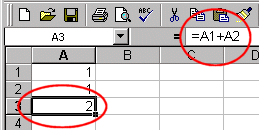Place the cursor in cell A3 and look at the Formula bar.

Subtraction

1. Press F5. The Go To dialog box will appear.
2. Type B1.
3. Press Enter.
4. The cursor should move to cell B1.
5. Type 5 in cell B1.
6. Press Enter.
7. Type 3 in cell B2.
8. Press Enter.
9. Type =+B1-B2 in cell B3.
10. Press Enter.
11. Note that cell B1 has been subtracted from B2 and the result is shown in cell B3.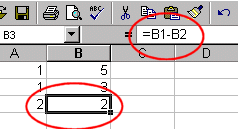Place the cursor in cell B3 and look at the Formula bar.

Multiplication

1. Hold down the Ctrl key while you press "g" (Ctrl-g). The Go To dialog box will appear.
2. Type C1.
3. Press Enter. You should now be in cell C1.
4. Type 2 in cell C1.
5. Press Enter.
6. Type 3 in cell C2.
7. Press Enter.
8. Type =C1*C2 in cell C3.
9. Press Enter.
10. Note that C1 is multiplied by C2 and the answer is displayed in C3.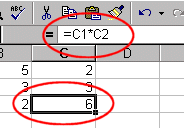Place the cursor in cell C3 and look at the Formula bar.

Division

1. Press F5.
2. Type D1.
3. Press Enter. You should now be in cell D1.
4. Type 6 in cell D1.
5. Press Enter.
6. Type 3 in cell D2.
7. Press Enter.
8. Type =D1/D2 in cell D3.
9. Press Enter.
10. Note that D1 is divided by D2 and the answer is displayed in cell D3.Place the cursor in cell D3 and look at the Formula bar.

Automatic Calculation

If you have automatic calculation turned on, Microsoft Excel recalculates the worksheet as you change cell entries. Let’s check to make sure automatic calculation is turned on.

Setting Automatic Calculation

1. Click on Tools, which is located on the Menu bar.
2. Press the down arrow key until Options is highlighted.
3. Press Enter.
4. Click on the Calculation tab if it is not in the front.
5. Select Automatic, if it is not already selected.
6. Click on OK.
Trying Automatic Calculation

Make the changes outlined below and note how Microsoft Excel automatically recalculates.

1. Hold down the Ctrl key while pressing Home (Ctrl-Home). This will move you to cell A1.
2. Type 2. Press the Tab key.
3. Note that the results shown in cell A3 have changed. The number in cell A1 has been added to the number in cell A2 and the results display in cell A3.
4. You should now be in cell B1.
5. Type 6. Press the Tab key.
6. Note that the results shown in cell B3 have changed. The number in cell B1 has been subtracted from the number in cell B2 and the results display in cell B3.
7. You should now be in cell C1.
8. Type 4. Press the Tab key.
9. Note that the results shown in cell C3 have changed. The number in cell C1 has been multiplied by the number in cell C2 and the results display in cell C3.
10. You should now be in cell D1.
11. Type 12. Press the Tab key.
12. Note that the results shown in cell D3 have changed. The number in cell D1 has been divided by the number in cell D2 and the results display in cell D3.
Formatting Numbers

You can format the numbers you enter into Microsoft Excel. You can add commas to separate thousands, specify the number of decimal places, place a dollar sign in front of the number, or display the number as a percent in addition to several other options.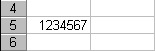Before formatting.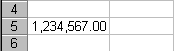After formatting.

1. Move the cursor to cell A5.
2. Type 1234567.
3. Press Enter.
4. Move the cursor back to cell A5.
5. Click on Format, which is located on the Menu bar.
6. Press the down arrow key until Cells is highlighted.
7. Press Enter.
8. Click on the Number tab, if it is not in the front.
9. Click on Number in the Category box.
10. Type 2 in the Decimal Places box. This will cause the number to display with two decimal places.
11. Place a checkmark in the Use 1000 Separator box. This will cause thousands to be separated with commas.
12. Click on OK.
Adding a Dollar Sign to the Numeric Entry
1. Move the cursor to cell A5.
2. Click on Format, which is located on the Menu bar.
3. Press the down arrow key until Cells is highlighted.
4. Press Enter.
5. Click on the Number tab, if it is not in the front.
6. Click on Currency in the Category box.
7. Make sure there is a "\$" in the Symbol box.
8. Click OK.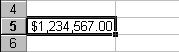Alternate Method – Formatting Numbers by Using the Toolbar1. Move the cursor to cell A6.
2. Type 1234567.
3. Press Enter.
4. Move the cursor back to cell A6.
5. Click twice on the Increase Decimal icon to change the number format to two decimal places. Clicking on the Decrease Decimal icon decreases the decimal places.
6. Click once on the Comma Style icon to add commas to the number.
7. To change the number to a currency format, click on the Currency Style format.
8. Move the cursor to cell A7.
9. Type .35 (note the decimal point).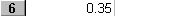1. Press Enter.
2. Move the cursor back to cell A7.
3. Click on the Percent Style icon to turn .35 to a percent.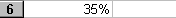When you perform mathematical calculations in Microsoft Excel be careful of precedence. Calculations are performed from left to right, with multiplication and division performed before addition and subtraction.

1. Move to a new worksheet by clicking on Sheet2, which is located in the lower left corner of the screen.
2. Go to cell A1.
3. Type =3+3+12/2*4.
4. Press Enter.
Note: Microsoft Excel divided 12 by 2, multiplied the answer by 4, added 3, and then added another 3. The answer 30 displays in cell A1.

To change the order of calculation, use parentheses. Microsoft Excel will calculate the information in parentheses first.

1. Double-click in cell A1.
2. Edit the cell to read =(3+3+12)/2*4.
3. Press Enter.
Note: Microsoft Excel added 3 plus 3 plus 12, divided the answer by 2, and multiplied the result by 4. The answer 36 appears in cell A1.

Microsoft Excel records cell addresses in formulas in three different ways, called absolute, relative, and mixed. The way a formula is recorded is important when you copy it.

With relative cell addressing, when you copy a formula from one area of the worksheet to another, Microsoft Excel records the position of the cell relative to the cell that originally contained the formula. The following exercises demonstrate:

Creating the Formula

1. Press F5.
2. Type A7. Press Enter.
3. Type 1. Press Enter.
4. Type 1. Press Enter.
5. Type 1. Press Enter.
6. Press F5.
7. Type B7. Press Enter.
8. Type 2. Press Enter.
9. Type 2. Press Enter.
10. Type 2. Press Enter.
11. Press F5.
12. Type A10.
13. Press Enter.
In addition to typing a formula as we did in Lesson 2, we can also enter formulas using the Point mode. When you are in the Point mode you can enter a formula either by clicking on a cell with your mouse or by using the arrow keys.
1. You should be in cell A10.
2. Type =.
3. Use the up arrow key to move to cell A7. Note that the word "Ready" in the lower right corner of the screen changes to "Point."
4. Type +.
5. Use the up arrow key to move to cell A8.
6. Type +.
7. Use the up arrow key to move to cell A9.
8. Press Enter.
9. Look at the formula bar while in cell A10. Note that the formula you entered is recorded in cell A10.

You can copy entries from one cell to another cell. To copy the formula you just entered, follow the steps outlined below:

1. You should be in cell A10.
2. Click on Edit, which is located on the Menu bar.
3. Press the down arrow key until Copy is highlighted.
4. Press Enter. Moving dotting lines will appear around cell A10. These dotted lines indicate the cells to be copied.
5. Press the Tab key once. This should move you to cell B10.
6. Click on Edit, which is located on the Menu bar.
7. Press the down arrow key until Paste is highlighted.
8. Press Enter. The formula in cell A10 should be copied to cell B10.
9. Press Esc to exit the Copy mode.
Compare the formula in cell A10 with the formula in cell B10 (while in the respective cell look at the formula bar). They are the same except the formula in cell A10 sums the entries in column A and the formula in cell B10 sums the entries in column B. The formula was copied in a relative fashion.

Before proceeding with the next exercise, we must copy the information in cells A7 to B9 to cells C7 to D9. This time we will copy by using the Formatting toolbar.

Copying by Using the Formatting Toolbar

1. Highlight cells A7 to B9. Place the cursor in cell A7. Press F8. Press the down arrow key twice. Press the right arrow key once. A7 to B9 should be highlighted.
2. Click on the Copy icon, which is located on the Formatting toolbar.
3. Use the arrow key to move the cursor to cell C7.
4. Click on the Paste icon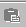, which is located on the Formatting toolbar.
5. Press Esc to exit the Copy mode.

An absolute cell address refers to the same cell, no matter where you copy the formula. You make a cell address an absolute cell address by placing a dollar sign in front of both the row and column identifiers. You can do this automatically by using the F4 key. To illustrate:

1. Move the cursor to cell C10.
2. Type =.
3. Use the up arrow key to move to cell C7.
4. Press F4. Dollar signs should appear before the C and before the 7.
5. Type +.
6. Use the up arrow key to move to cell C8.
7. Press F4.
8. Type +.
9. Use the up arrow key to move to cell C9.
10. Press F4.
11. Press Enter.
12. The formula is recorded in cell C10.
Copying by Using the Keyboard Shortcut

Now copy the formula from C10 to D10. This time, copy by using the keyboard shortcut.

1. Your cursor should be in cell C10.
2. Hold down the Ctrl key while you press "c" (Ctrl-c). This copies the contents of cell C10.
3. Press the Tab key once.
4. Hold down the Ctrl key while you press "v" (Ctrl-v). This will paste the contents of cell C10 in cell D10.
Compare the formula in cell C10 with the formula in cell D10. They are exactly the same. The formula was copied in an absolute fashion. Both formulas should add up column C.

You use mixed cell addressing to reference a cell that is part absolute and part relative. You can use the F4 key.

1. Move the cursor to cell E1.
2. Type =.
3. Press the up arrow key once.
4. Press F4.
5. Press F4 again. Note that the column is relative and the row is absolute.
6. Press F4 again. Note that the column is absolute and the row is relative.
7. Press Esc.
Reference Operators

Reference operators are helpful when referring to a cell or group of cells. Two types of reference operator are range and union.

A range reference refers to all the cells between and including the reference. A range reference consists of two cell addresses separated by a colon. The reference A1:A3 includes cells A1, A2, and A3. The reference A1:C3 includes A1, A2, A3, B1, B2, B3, C1, C2, and C3.

A union reference includes two or more references. A union reference consists of two or more cell addresses separated by a comma. The reference A7,B8,C9 refers to cells A7, B8, and C9.

Functions

Microsoft Excel has a set of prewritten formulas called functions. Functions differ from regular formulas in that you supply the value but not the operators, such as +, -, *, or /. The SUM function is used to calculate sums. When using a function, remember the following:

Use an equals sign to begin a formula

Specify the function name

Enclose arguments within parentheses

Use a comma to separate arguments

Here is an example of a function: =SUM(2,13,10,67)In this function: The equals sign begins the function

SUM is the name of the function

2, 13, 10 and 67 are the arguments

Parentheses enclose the arguments

A comma separates each of the arguments

The SUM function adds the arguments together. In the exercises that follow, we will look at various functions.

Typing a Function

1. Click on Sheet3 located at the bottom of your window to move to a new worksheet:
2. Type 12 in cell B1.
3. Press Enter.
4. Type 27 in cell B2.
5. Press Enter.
6. Type 24 in cell B3.
7. Press Enter.
8. Type =SUM(B1:B3) in cell A4. Microsoft Excel sums cells B1 to B3.
Alternate Method – Entering a Function by Using the Menu
1. Type 20 in cell C1.
2. Press Enter.
3. Type 30 in cell C2.
4. Press Enter.
5. Type 50 in cell C3.
6. Press Enter. Your cursor should be in cell C4.
7. Click on Insert, which is located on the Menu bar.
8. Press the down arrow key until Function is highlighted.
9. Press Enter.
10. Click on Math & Trig in the Function Category box.
11. Click on Sum in the Function Name box.
12. Click on OK.
13. Type C1:C3 in the Number1 entry field, if it does not automatically appear.
14. Click on OK.
15. Move to cell A4.
16. Type the word Sum.
17. Press Enter.
Calculating an Average

You can use the AVERAGE function to calculate an average from a series of numbers.

1. Move the cursor to cell A5.
2. Type Average.
3. Press the right arrow key.
4. Type =AVERAGE(B1:B3).
5. Press Enter. The average should appear.
Calculating Min

You can use the MIN function to find the lowest number in a series of numbers.

1. Move the cursor the cell A6.
2. Type Min.
3. Press the right arrow key.
4. Type = MIN(B1:B3).
5. Press Enter. The lowest number in the series, which is 12, should appear.
Calculating Max

You can use the MAX function to find the highest number in a series of numbers.

1. Move the cursor the cell A7.
2. Type Max.
3. Press the right arrow key.
4. Type = MAX(B1:B3).
5. Press Enter. The highest number in the series, which is 27, should appear.
Saving Your File and Closing Microsoft Excel

This is the end of Lesson Three. Save your file and close Microsoft Excel.

1. Click on File, which is located on the Menu bar.
2. Press the down arrow key until Save is highlighted.
3. Press Enter.
4. Type lesson3.xls in the filename field.
5. Click on Save.
6. Click on File, which is located on the Menu bar.
7. Press the down arrow key until Exit is highlighted.
8. Press Enter.